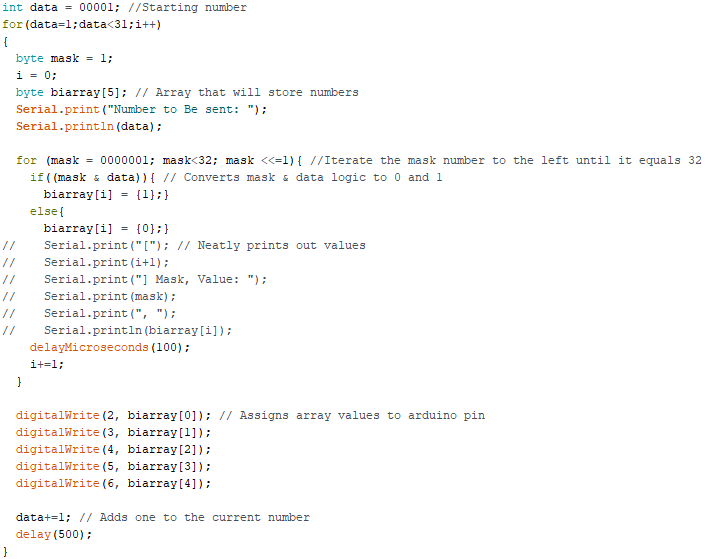ENGR 361 2020 Fall

Lab 2 Basic Arduino Code
Nic Theobald
nstheobald@fortlewis.edu

Basic Arduino Code
Introduction
• This lab covers some basic Arduino code such as&
•   If Statements
• For and While Loops
• Data Types
• Switch Statements
• Serial Communication
• Logic
• Pin Control

Materials

 Materials x Computer 1 Arduino IDE 1 Your Brain 1 Some Patience 1

Abbreviated Methods

The first step in this lab was to get the Arduino to communicate with the PC. To get it to do this, a program was created that instructed the Arduino to send print to the serial monitor. The lab ran through some basic algebra and loops and ended with representing a binary number in LEDs. I added another loop that counts up from zero and displays it on the LEDs.

Results

A communication test was run to get the Arduino to send print to the serial monitor.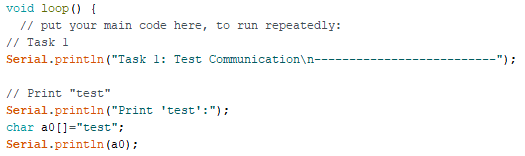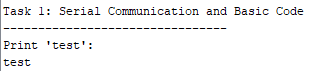Figure 1: Test print.

Boolean and basic mathematics were also tested.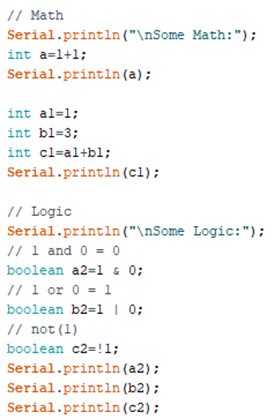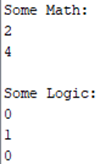Figure 2: Mathematics.

The if statement was used to test the value of an integer.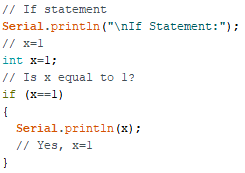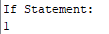Figure 3: Testing the value of x.

Different loops were used to iterate through a range of numbers and perform certain tasks. First is the for loop. It iterates through the range of numbers while performing a task.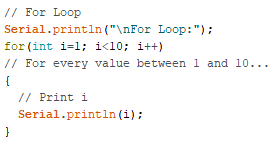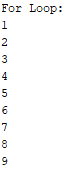Figure 4: Looping through integers between 0 and 10.

Of course, an if statement can be incorporated into the do part of the for loop& In this case, multiplying odd numbers by 100.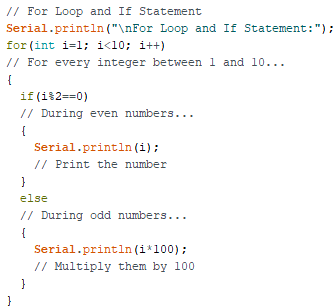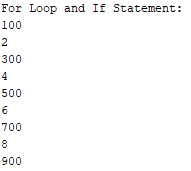Figure 5: Looping through integers between 0 and 10 and multiplying odd numbers by 100.

The while loop runs its code so long as its condition is met.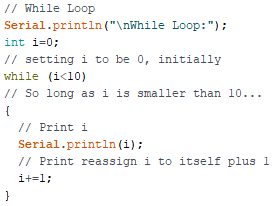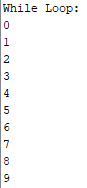Figure 6: Iterating when integers are below 10.

Switch statement is similar to the If statement. If this = this, do that&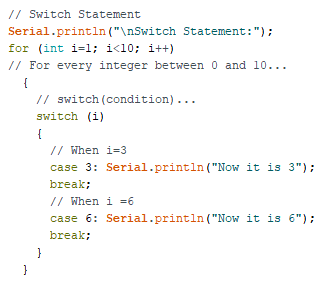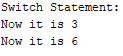Figure 7: Performing a specific task when the condition is satisfied.

The do while loop was also investigated. The idea of the do while loop was new to me, but seems to be very useful& The idea is that it checks its condition after running the code it contains& Like in my example, I was initially 0. I, being zero immediately broke the while loop but the do while loop didnt care. It ran the code, which then reassigned I to be 1. Therefore, allowing the do while loop to keep its code.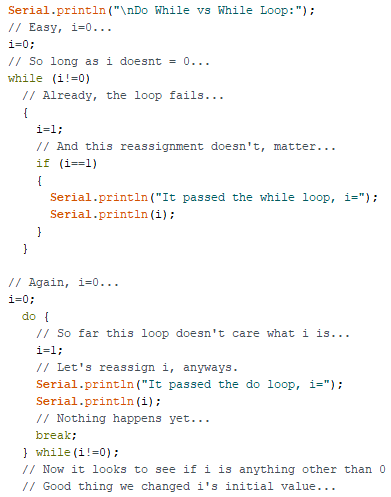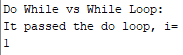Figure 8: Comparison of the do while and the while loop&

The code can also convert Hex Numbers to decimal&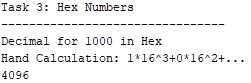Figure 9: Hand calculation of 1000 in HEX

Converting numbers into hex and confirming with Arduino.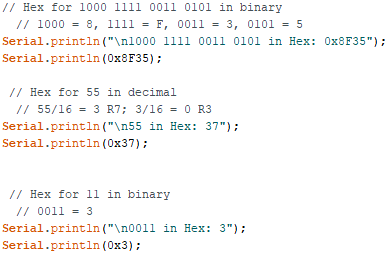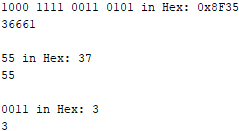Figure 10: Converting number to hex.

Converting hex into binary and confirming with Arduino.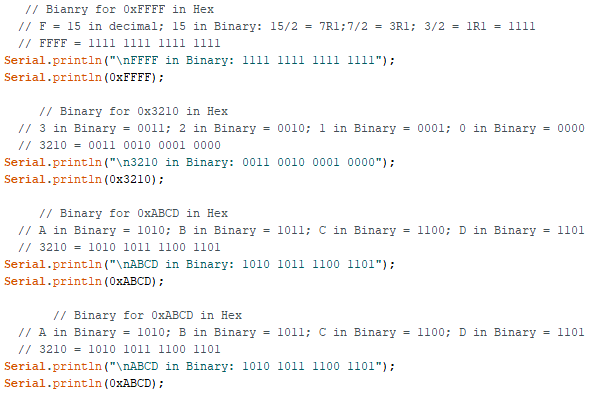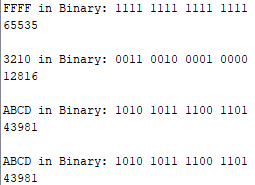Figure 11: Converting hex to binary.

Convert binary number into LED combination. The following code uses a bit mask to iterate through the given number (using & logic) to assign the binary number to pins on the Arduino.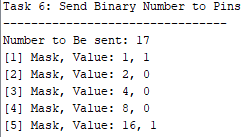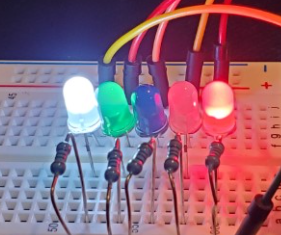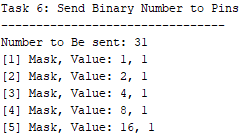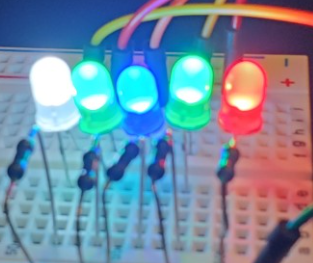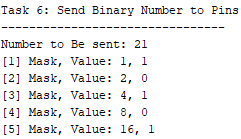Figure 12: Converts binary number into led combinations.